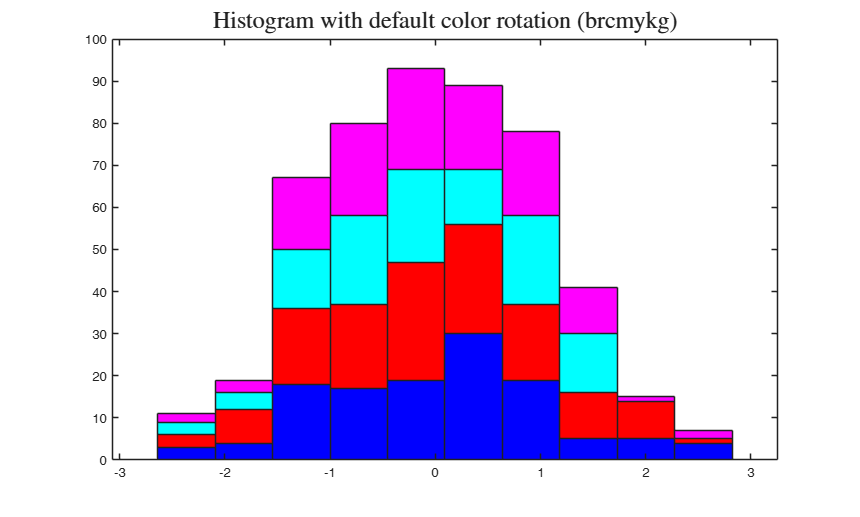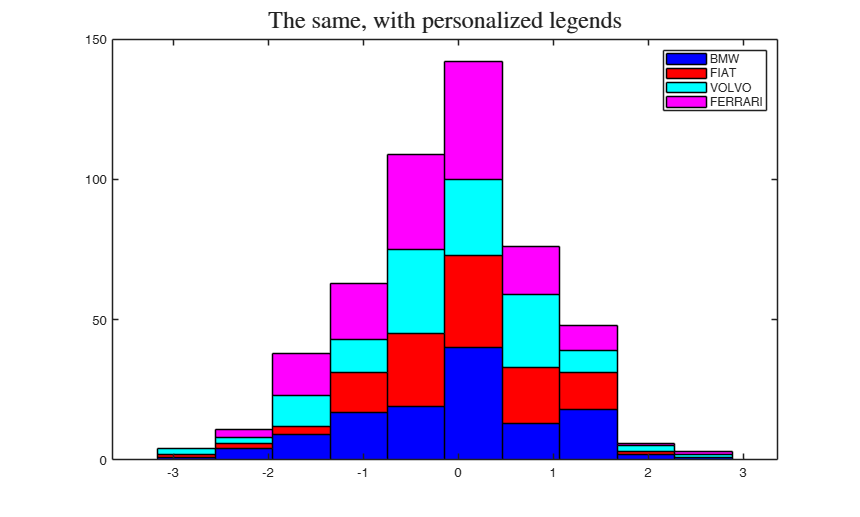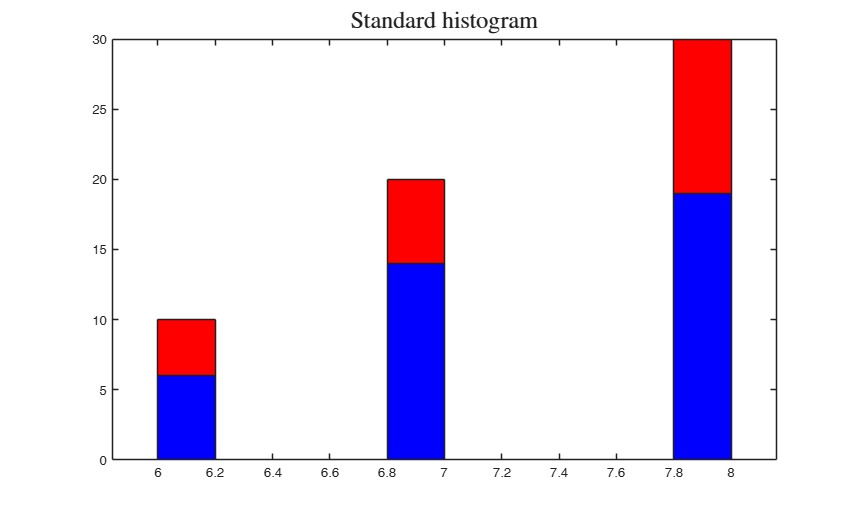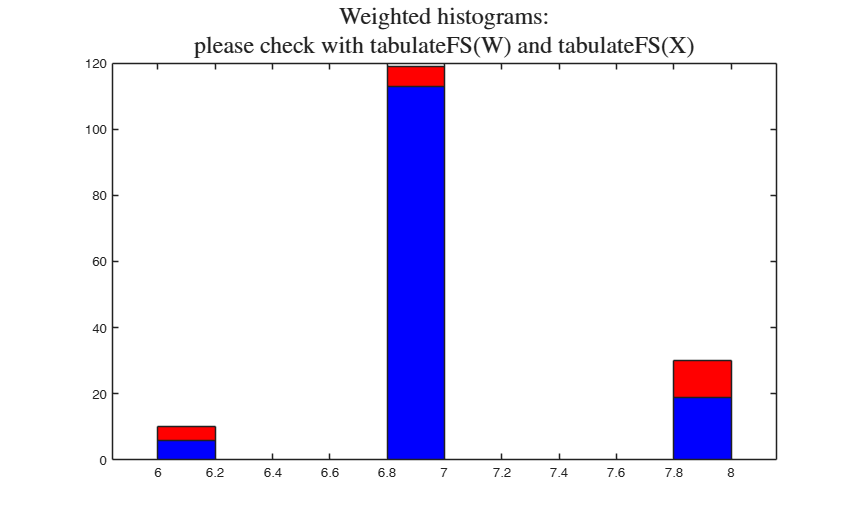# histFS

histFS plots a histogram with the elements in each bin grouped according to a vector of labels.

## Syntax

• ng=histFS(y,nbins,gy)example
• ng=histFS(y,nbins,gy,gylab)example
• ng=histFS(y,nbins,gy,gylab,ax)example
• ng=histFS(y,nbins,gy,gylab,ax,barcolors)example
• ng=histFS(y,nbins,gy,gylab,ax,barcolors,W)example
• [ng, hb]=histFS(___)example

## Description

 ng =histFS(y, nbins, gy) An example with 4 groups.

 ng =histFS(y, nbins, gy, gylab) The same histogram is now plotted with different legends.

 ng =histFS(y, nbins, gy, gylab, ax) The same histogram is now plotted with different colors.

 ng =histFS(y, nbins, gy, gylab, ax, barcolors) Apply to the grouped histogram the legends of a different plot.

 ng =histFS(y, nbins, gy, gylab, ax, barcolors, W) Example with personalised clickable legends.

 [ng, hb] =histFS(___) Example of weighted histogram.

## Examples

expand all

###An example with 4 groups.

close all;
y = randn(500,1);
% four groups
groups = randi(4,500,1);
% number of bins
nbins = 10;
[ng, hb] = histFS(y,nbins,groups);
title('Histogram with default color rotation (brcmykg)','interpreter','latex','FontSize',18);###The same histogram is now plotted with different legends.

figure;
y = randn(500,1);
groups = randi(4,500,1);
nbins = 10;
[ng, hb] = histFS(y,nbins,groups,{'BMW','FIAT','VOLVO','FERRARI'});
title('The same, with personalized legends','interpreter','latex','FontSize',18);###The same histogram is now plotted with different colors.

figure;
y = randn(500,1);
groups = randi(4,500,1);
nbins = 10;
[ng, hb] = histFS(y,nbins,groups,{'BMW','FIAT','VOLVO','FERRARI'},gca,'kgbr');
title('FERRARI must be red! Color sequence changed to kgbr','interpreter','latex','FontSize',18);### Apply to the grouped histogram the legends of a different plot.

Create a scatterplot

close all;
y = randn(500,1);
groups = randi(4,500,1);
nbins = 10;
hs = gscatter(1:numel(y),y,groups);
hfs = gcf;                              % get the handle of the scatterplot
has = get(hfs,'CurrentAxes');           % it is the same as has = gca
hlegends  = get(has,'Children');        % get the legend entries
getleg = get(hlegends,'DisplayName');   % get the names of the legend entries
getcol = get(hlegends,'Color');         % get the color of the legend entries
getcolm = cell2mat(getcol);             % arrange the RGB vectors into a matrix
figure;
[ng, hb] = histFS(y,nbins,groups,getleg,gca,getcolm);
title('Color sequence is taken from the scatterplot','interpreter','latex','FontSize',18);

### Example with personalised clickable legends.

y = randn(500,1);
groups = randi(4,500,1);
nbins = 10;
myleg = {'my group 1' 'my group 2' 'my group 3' 'my group 4' };
[ng, hb] = histFS(y,nbins,groups,myleg);
title('Example with personalised clickable legends','interpreter','latex','FontSize',18);

###Example of weighted histogram.

close all;clear all;
X = crosstab2datamatrix([10 20 30]); X=X(:,2);
X1 = 444*ones(60,1); X1(40:60)= 999; X1 = shuffling(X1);
X = X+5;
if verLessThan('matlab','8.5')
groups = num2str(X1);
else
groups = categorical(X1);
end
W = ones(size(X,1),1); W(12) = 100;
histFS(X,10,groups,[],[],[],W);
title({'Weighted histograms:' , 'please check with tabulateFS(W) and tabulateFS(X)'},'interpreter','latex','FontSize',18);
figure;
histFS(X,10,groups);
title('Standard histogram','interpreter','latex','FontSize',18);
disp('tabulateFS(X)');
tabulateFS(X);
disp('tabulateFS(W)');
tabulateFS(W);
tabulateFS(X)
6       10     16.67%
7       20     33.33%
8       30     50.00%
tabulateFS(W)
1       59     98.33%
100        1      1.67%## Input Arguments

### y — vector of n elements to bin. Vector.

Vector which contains the elements which have to be binned.

Data Types: single| double

### nbins — the number of bins. Scalar.

The elements of of input vector y are binned into nbins equally spaced containers.

Data Types: single| double

### gy — identifier vector. Vector.

Vector of n numeric identifiers for the group of each element in y. If there are k groups in y, unique(gy) = k.

Data Types: single| double

### gylab — legend labels. String | cell of strings.

Legend labels identifying the groups of each element in y.

length(gylab) = length(unique(gy)) must hold.

If not specified, gylab is set to '' and legends are not displayed.

If gylab = {}, default legend labels are generated.

If gylab is a cell of strings of length 3, e.g. gylab = {'G1' 'G2' 'G3'}, such strings are used for the legends.

Example: {'G1' 'G2'} 

Data Types: cell array of strings or char

### ax — plots into ax instead of gca. Axis handle.

The axis handle where to plot the grouped histogram (e.g. a traditional histogram plot to be superimposed). Default is gca.

Example: gca 

Data Types: graphics handle

### barcolors — colors of the bars. char or matrix.

Vector containing the strings of the colors to use (e.g. 'rgy') or RGB matrix of the colors used for the groups (e.g. [1 0 0; 0 0 1]). If the number of colors supplied is smaller than the number of groups the program displays an error.

Example: 'rgy' 

Data Types: character or numeric matrix

### W — Weights. Vector.

Vector which contains optional weights associated to the elements of y.

Example: [10 20 3000] 

Data Types: numeric vector

## Output Arguments

### ng —number of elements in each container for each group.  Matrix

A matrix with a column for each group and a row for each bin. In ng, column i contains the the number of elements of group i in each bin.

For example ng(2,3) contains the number of elements belonging to the third group included into the second container.

### hb —Handles to the barseries objects. Bar array handles. Vector. A vector

Tufte E.R. (1983), "The visual display of quantitative information", Graphics Press, Cheshire.